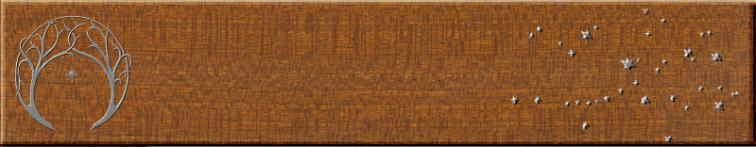Ross Sackett's amateur telescope makingRoss Sackett's amateur telescope making
Articles
 Scaling Single-Pole Telescopes
September 23, 2006

Background: For maximum stiffness, you can’t beat a truss or tube scope.  However, both suffer from the
drawbacks of bulk and complexity.  Many telescope makers have attempted designs with from one to four
cantilevered poles to simply their scopes and make them more portable.  The telescope maker much choose the
number, diameter, length, and cross-section of the pole(s).  What are the considerations?

Method: I used beam equations in an Excel file to estimate the flexure of various cantilevered poles and
configurations.

The maximum flexure of a cantilevered pole (when the pole is horizontal and loaded at the free end) is given by the
equation

1        WL^3
Ymax =   ---      -------
3        EI

where W is the load in lbs, here equivalent to the weight of the UTA; L is the unsupported length of the pole in
inches; E is the modulus of elasticity of the material in psi, here assumed to be 10,000,000 psi for aluminum; and I
is the section modulus for the pole in in^4.

The section modulus for a thin-walled tube with a circular cross-section is

I = π r3 t

where r is the “average” radius of the tube in inches (approximately equal to the average of the outer and inner
diameters) and t is the wall thickness in inches.  This can be re-expressed in “average” diameters as

π D^3 t
I = -------------
8

An alternative formula is

π (D^4 – d^4)
I =    ---------------
64

where D is the outer diameter and d is the inner diameter.  For a thin-walled square section tube

W^4 – w^4
I =    ------------
12

where W is the outer width and w is the inner width of the tube.

Conclusions
1.  Choose a large-diameter pole
.   Wider poles are much better than narrower ones.  The stiffness of a pole
increases with the cube of the diameter.  If you double the diameter of the pole (maintaining the same wall
thickness and length) the flexure for a given load is reduced by a factor of 8.  Even a modest increase in diameter
of only 25% cuts the flexure in half.  It is important that poles be as wide as possible.

2. One pole is best.   One pole is much better and two or more.  Comparing poles of same length and wall
thickness, one pole 2” in diameter has the same weight as two 1” poles or four 0.5” poles.  Even though the load is
spread over more members, the single pole has a huge advantage: it is four times as stiff as the two pole
configuration and over twenty times as stiff as the four pole arrangement.  One big pole is much stiffer than two or
more poles.  The “Alice” and Plettstone scopes would both be stiffer and lighter with single poles.

3. Keep the length as short as practical.  Shorter poles are much stiffer than longer poles.  The flexure of a pole
increases with the cube of its length.  Thus, a slight increase in the length of the pole results in a large increase in
flexure: if you double the length, the pole will deflect eight times as much.  This is true even for modest increases in
length: an increase in length of only 25% results doubles the flexure at a given load.  In my designs it is best to
keep the bracket that holds the tube as long as practical to keep the tube run short.

4. Use round tubes.  Round tubes are stiffer in bending and twisting than square tubes.  For a given cross-
sectional area and wall thickness, a square tube bends about 20% more than a circular tube.  It also is far less
resistant to torsion: a circular tube is about 1.9 times as resistant to twisting.

Excel table:
Single-tube telescopes: beam calculations

No. tubes        OD        ID        L        Total W        Supported W        E        I        Ymax        Factor
Comparison of tubes of different diameter
1        3        2.875        36        4        4        10000000        0.6224        0.009994855        28.71
1        2.5        2.375        36        4        4        10000000        0.3557        0.017489903        50.24
1        2        1.875        36        4        4        10000000        0.1787        0.034812073        100
1        1.5        1.375        36        4        4        10000000        0.073        0.085165352        244.6
1        1        0.875        36        4        4        10000000        0.0203        0.306243551        879.7
1        0.5        0.375        36        4        4        10000000        0.0021        2.966187541        8521

Comparison of different numbers of tubes with same total cross-sectional area and weight
1        2        1.875        36        4        4        10000000        0.1787        0.034812073        100
2        1        0.875        36        4        2        10000000        0.0203        0.153121776        439.9
4        0.5        0.375        36        4        1        10000000        0.0021        0.741546885        2130
8        0.25        0.125        36        4        0.5        10000000        0.0002        4.325690163        12426

Comparison of tubes of different length
1        2        1.875        18        4        4        10000000        0.1787        0.004351509        12.5
1        2        1.875        36        4        4        10000000        0.1787        0.034812073        100
1        2        1.875        45        4        4        10000000        0.1787        0.06799233        195.3
1        2        1.875        72        4        4        10000000        0.1787        0.278496584        800
1        2        1.875        90        4        4        10000000        0.1787        0.54393864        1562

Comparison of round and square tubes with same cross-sectional area
Round tube
1        2        1.875        36        4        4        10000000        0.1787        0.034812073        100
Square tube
1        1.5985        1.4735        36        4        4        10000000        0.1512        0.041130616        118.2

Comparison of round and square tubes in torsion
A seamless round tube is about 1.87X as resistant to twisting
as a square tube of the same weight and cross-sectional area
(James Ambrose (2002) Simplified Mechanics and Strength of Materials, Wiley, P. 45)